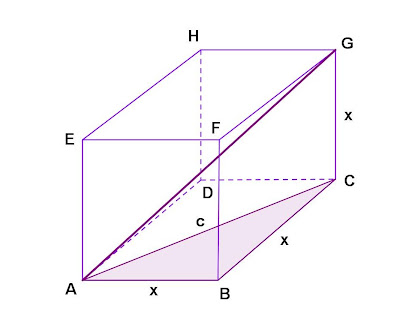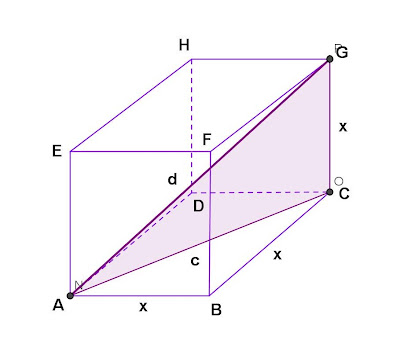## Monday, January 7, 2013

### Volume - Cube, Given Diagonal

Category: Solid Geometry, Plane Geometry

"Published in Newark, California, USA"

A diagonal of a cube joints two vertices not in the same face. If the diagonals are 4√3 cm. long, what is the volume?

Solution:

To visualize the problem, let's draw the figure as followsPhoto by Math Principles in Everyday Life

We know that all sides of a cube are equal because all faces of a cube are square. All sides of a cube are perpendicular to each other. A diagonal is a line segment that connects the two opposite vertices of a cube. There are 4 equal diagonals in a cube: AG, CE, BH, and FD.

How do you get the length of a diagonal of a cube if one side of a cube is given? Here's the procedure in getting the length of a diagonal of a cube as followsPhoto by Math Principles in Everyday Life

By Pythagorean Theorem

After we get the diagonal of a base, we can finally get the diagonal of a cube as followsPhoto by Math Principles in Everyday Life

By Pythagorean Theorem

The length of a diagonal of a cube is equal to the length of a side of a cube times square root of three. From the given word problem that if the length of a diagonal of a cube is 4√3 cm., then the length of a side of a cube will be

Finally, we can get the volume of a cube as follows